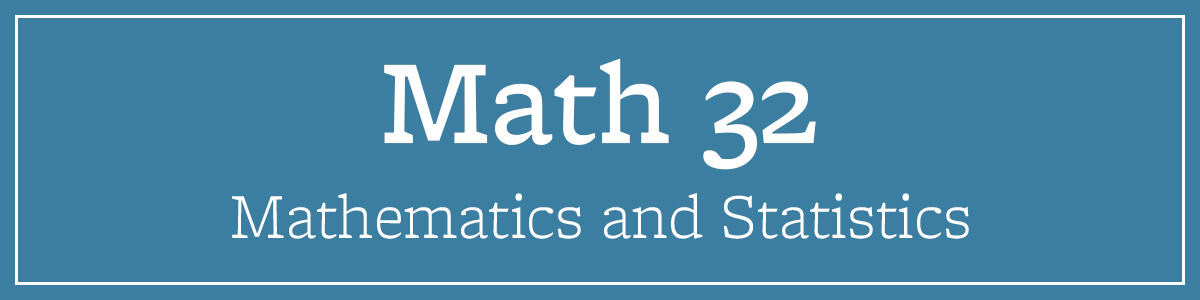# Math 32Pre-Calculus

## Course Description

Polynomial and rational functions, exponential and logarithmic functions, trigonometry and trigonometric functions. Complex numbers, fundamental theorem of algebra, mathematical induction, binomial theorem, series, and sequences.